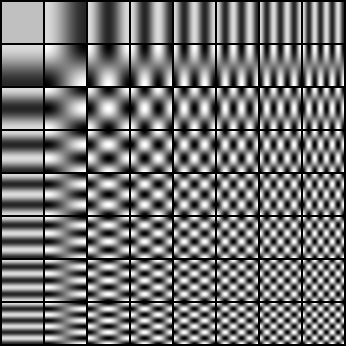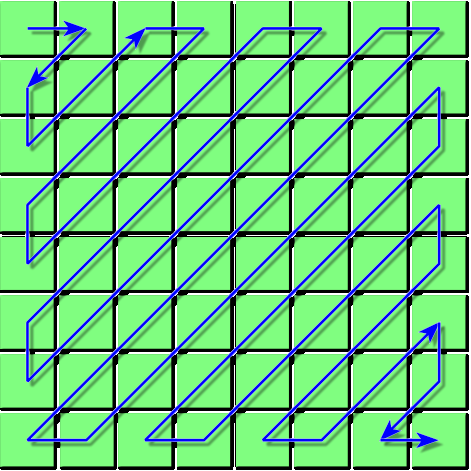Warning
This page is located in archive.

# 2. Computing local invariant description

To compute a local description invariant to geometric and photometric transformations, the neighborhood of the feature points needs to be normalized to undo the effects of transformations. After elimination of these deformations, the invariant description can be computed.

As we already know, the task of the detector is to repeatedly find the shape of the neighborhood of feature points in such a way that the detected points are co-variant with a desired class of transformations (geometric or photometric). For instance, the basic version of Harris or Hessian detector detects points which are co-variant with translation (if you shift the image, points are shifted too). Hessian in the scale-space or DoG detector adds information about the scale of the region and therefore points are co-variant with similarity transformation up to an unknown rotation (detected points have no orientation assigned). Affine co-variant detectors like MSER, Hessian-Affine or Harris-Affine are co-variant up to an affine transformation. The following text describes how to handle geometric information from the point neighbourhood and how to use it for normalization. Depending on the amount of information that a detector gives about a features, the descriptions can be invariant to translation, similarity, affine or perspective transformation.

## Geometric normalization

Geometric normalization is a process of geometric transformation of feature neighborhood into a canonical coordinate system. Information about geometric transformation will be stored in the form of “frame” – a projection of the canonical coordinate system into the neighborhood of a feature point (or region) in the image. The frame will be represented by a 3×3 matrix A, the same as in the first lab. The transformation matrix A is used to obtain a “patch” – a small feature neighborhood in the canonical coordinate system. All other measurements on this square patch of original image are now invariant to desired geometric transformation.

For the construction of a transformation A, we use the geometric information about position and shape of point neighborhood:

1. For rotation- and translation-covariant transformation, we have coordinates x,y and angle $\alpha$. The scale s (size of the frame) is fixed (manually) for all points.
$$A=\underbrace{\left[\begin{array}{ccc} s & 0 & x\\ 0 & s & y\\ 0 & 0 & 1\\ \end{array}\right]}_{A_{norm}} \left[\begin{array}{ccc} cos(\alpha) & sin(\alpha) & 0\\ -sin(\alpha)& cos(\alpha) & 0\\ 0 & 0 & 1\\ \end{array}\right]$$
2. For similarity-covariant point, we have coordinates x,y scale $\sigma$ and angle $\alpha$.
$$A=\underbrace{ \left[\begin{array}{ccc} \sigma & 0 & x\\ 0 & \sigma & y\\ 0 & 0 & 1\\ \end{array}\right]}_{A_{norm}} \left[\begin{array}{ccc} cos(\alpha) & sin(\alpha) & 0\\ -sin(\alpha)& cos(\alpha) & 0\\ 0 & 0 & 1\\ \end{array}\right]$$
3. For affine-covariant point, we can use three points to which the points from the canonical coordinate system are projected (as in the first lab). We can also use a known position, partial affine transformation (a 2×2 submatrix) and the residual rotation (angle $\alpha$) to construct the matrix A:
$$A=\underbrace{ \left[\begin{array}{ccc} a_{11} & a_{12} & x\\ a_{21} & a_{22} & y\\ 0 & 0 & 1\\ \end{array}\right]}_{A_{norm}} \left[\begin{array}{ccc} cos(\alpha) & sin(\alpha) & 0\\ -sin(\alpha)& cos(\alpha) & 0\\ 0 & 0 & 1\\ \end{array}\right]$$

Many detectors (all from the last lab) detect points (frames), which are similarity- or affine- covariant up to an unknown rotation (angle $\alpha$). For instance, the position and scale give us a similarity co-variant point up to unknown rotation. Similarly, the matrix of second moments and the centre of gravity give us five constraints for an affine transformation and only the rotation remains unknown. To obtain a fully similarity- or affine-covariant point, we need to define orientation $\alpha$ from a partially geometrically normalized point neighborhood.

The normalization including orientation estimation has to be done in two steps. In the first step, the patch invariant to translation, scale and partial affine transformation is created. On this normalized patch, the dominant gradient is estimated. Both transformation are then used together in the transformation matrix A.

To estimate the orientations of the dominant gradients on the partially normalized regions (using $A_{norm}$), the gradient magnitudes and orientations are estimated for each region and a histogram of these gradients is computed. While we assume a random rotation of the region, it is necessary to compute gradients only in circular neighborhood. This can be done by weighting with a window-function (e.g. Gaussian) or with a condition like $(x-x_{center})^2+(y-y_{center})^2 < (ps/2)^2$, where $ps$ patch edge length. Otherwise the content of the corners would influence the orientation estimate undesirably. The orientations of gradients are weighted by their magnitudes. This means that a “stronger” gradient brings more to the corresponding bin of the histogram. For improved robustness, we can use linear interpolation to vote into the closest neighboring bins. At the end, the histogram is filtered with a 1D Gaussian and the maximum is found. For a more precise localization it is possible to fit a parabola into the maximum neighbourhood and to find the orientation with a precision over 360/(number of bins) degrees.

• Write a function angle=dom_orientation(img) for dominant orientation estimation in a normalized patch. The input img is a partially normalized patch of the image (ps x ps matrix of doubles), the output is the angle measured from the x-axis of the image in clock-wise direction (angle for vector (x,y) can be computed with function atan2(y,x)).

Now we are able to write the functions for geometric normalization of feature point neighborhoods with dominant orientations:

• for a known position, write a function pts=transnorm(img,x,y,s,opt), where img is the input image, x,y are row vectors of point coordinates and s is a scalar with the fixed point scale (the same value for all feature points).
• for a known position and scale, write a function pts=simnorm(img,x,y,s,opt), where img is the input image, x,y are row vectors of point coordinates and s is a row vector with the scales of all points (see the output of the sshesian function).
• for a known position and partial affine transformation, write a function pts=affnorm(img,x,y,a11,a12,a21,a22,opt), where img is the input image, x,y are row vectors of points coordinates and a11,a12,a21,a22 are row vectors with the partial affine transformation elements.

Input parameter opt is a structure containing normalization parameters:

opt.ps - size of the patch (edge length)
opt.ext - size of the neighborhood in the canonical coordinate system

The output pts is a one-dimensional array of structures, containing for each point: coordinates - x,y, elements of the 2×2 partial affine transformation submatrix - a11,a12,a21,a22, and the normalized image patch (ps x ps matrix of type double) - patch. Use the hierarchy of these functions at implementation - a simpler function can be used by more complex ones. The resulting patch and the patch for dominant orientation estimation are computed using function affinetr.

Here's a pseudocode for normalization including orientation estimation:

% ... for each point ...
A = create_matrix_A_without_rotation(x(id),y(id),...);
% orientation estimation
tmp = affinetr(img, A, opt.ps, opt.ext);
angle = dom_orientation(tmp);
% final A, dominant orientation to angle zero
R = rotation_2x2(-angle); A(1:2,1:2) = A(1:2,1:2)*R;
% create output
pts(id).x=x(id); pts(id).y=y(id);
pts(id).a11=A(1,1);
...
pts(id).patch=affinetr(img, A, opt.ps, opt.ext);
For the sake of clarity only the orientation of the strongest gradient is used for normalization in this task.

## Photometric normalization

The goal of photometric normalization is to suppress the scene changes caused by illumination changes. The easiest way of normalization is to expand the intensity channel into whole intensity range. We can also expand the intensity channel such that(after transformation) the mean intensity is at half of the intensity range and the standard deviation of intensitiescorresponds to it's range (or better). In case we want to use all three color channels, we can normalize each channel separately.

• Write a function ptsn=photonorm(pts), which for each point in the array of structures pts photometrically normalizes the patch of the image (field patch) such that the mean intensity value will be 0.5 and the standard deviation will be 0.2. Values outside the range <0,1> will be set to 0 or 1 respectively. Function returns an array of structures ptsn similar to the input array of structures pts, with modified patches in the fields patch an with the original mean values and standard deviations in fields mean and std.

## Computing the description

On the geometrically and photometrically invariant image patch from the last step, any low dimensional description can be computed. It is clear that the process of normalization is not perfect and therefore it is desirable to compute a description which is not very sensitive to the residual inaccuracies. The easiest description of the patch is the patch itself. Its disadvantages are high dimensionality and sensitivity to small translations and intensity changes. Therefor we will try better descriptions too.

1. A description using the two-dimensional discrete cosine transformation(DCT). Coefficients of this integral transformation are computed by the dot product of image the patch with the so called DCT base functions. See image:The image patch is decomposes into individual “frequencies” in a way similar to the JPEG compression. Similarly to image compression, we chose a subset of these coefficients containing information about lower frequencies. Discarding higher frequencies brings robustness to high frequency noise and to small errors in geometric normalization. It can be seen from the image of base functions that the choice of DCT coefficients will be done in so called zig-zag order, where the the higher frequencies are added after the lower ones.
• Write a function dct=dctdesc(img,num_coeffs) computing the DCT transformation for input image patch img and returns num_coeffs coefficients in zig-zag order in variable 'dct'. Normalize the values such that all possible DCT coefficients belong to <0,1>. You can make use of the MATLAB function dct2.
2. (optional task) RGB histogram, RG histogram and the histogram of gradients are descriptions using global image patch properties. Color channels of the image (e.g. R, G, B) are quantized into bins after pre-processing and theit characteristics are then accumulated. The resulting description is a linear vector of values accumulated into bins. The so called RG chromacity color space has two components,
it is a ratio of red and green component in the image. The advantage of image patch histograms is their insensitivity to geometric normalization errors, the disadvanige is a lower distinctness.
• Write a function dxdy=ghistodesc(img,num_bins) computing the histogram of gradients, num_bins is the number of bins per channel, so the resulting dxdy description is a column vector with num_bins2 elements of the histogram (size of the gradient in dx - row, dy - column) joined by columns (matlab operator (:) ). Normalize the elements into range <0,1> (0 - no occurrence, 1 - occurres in all points of the patch).

## What should you complete?

You are supposed to complete functions transnorm.m, simnorm.m, affnorm.m, photonorm.m, dom_orientation.m, dctdesc.m together with all used non-standard functions you have created.

## Testing

To test your code, copy desc_test.zip and unpack it to direcotry in MATLAB paths (or put it into the directory with your code) and execute. Compare your results with ours.

courses/ucuws17/labs/03_2_cspond/start.txt · Last modified: 2017/01/13 18:12 by prittjam